# Multilabel Document Categorization, step by step example

This detailed guide explores an unsupervised and supervised learning two-stage approach with LDA and BERT to develop a domain-specific document categorizer on unlabeled documents.

By Saurabh Sharma, Machine Learning Engineer.Photo by Anete Lusina on pexels.

In a real-world scenario, documents that we encounter usually cover more than one topic. A topic is something that describes the meaning of a document concisely. For instance, let’s take one review from a garage service website — “The process of booking was simple, and the tire I bought was a good price. The only issue was that service took 35 minutes from arriving at the depot to leaving, which I felt was too long for a pre-arranged appointment.

In this review, there are numerous intuitions, such as — “ease_of_booking”, “tyre_price”, “service_duration” — that we can call “topics.” It’s been a challenging task for researchers to churn out such topic clusters from a raw unstructured set of documents. I propose a two-step approach using LDA and BERT to build a domain-specific document categorizer that categorizes each document into a set of topic clusters from raw unlabelled document datasets.

My approach involves two main subtasks:

1. Unsupervised learning using LDA (Latent Dirichlet Allocation) to mine a set of topics from an unlabelled document dataset.
2. Supervised learning using BERT to build a muti-topic document categorizer.

Let’s begin…

## Data

I scrapped reviews of an online garage booking service website for this task and stored them into a CSV file.

```import numpy as np
import pandas as pd # data processing, CSV file I/O
import os
import re
## provide your own file path

```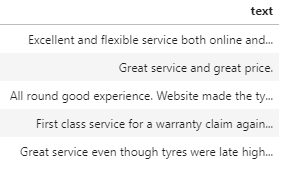Created by the author.

## Unsupervised learning using LDA

The following pre-processing steps were performed before training the garage booking service reviews using LDA.

### Data-cleaning

This module is the initial and crucial phase of the pre-processing, where the text data was cleaned by removing punctuation, stop words, non-ASCII values. Finally, the list of lemmatized tokens for each of the reviews is returned as an output.

```import spacy

```

The above lines of code will load the spacy model.

```def preprocess_text(text):
doc1 = nlp(text.lower())
preprocessed_txt = [str(token.lemma_) for token in doc1 if not       token.is_stop and not token.is_punct and not token.is_digit and token.is_ascii]
return preprocessed_txt
##making call to preprocess_text function
train_df['text'] = train_df.text.apply(preprocess_text)
## this cleaned data will act as our cleaned corpus
corpus_txt = train_df['text']

```

### Feature Engineering

Feature engineering involved the following two crucial modules:

1. Getting bigrams and trigrams tokens for a list of review documents and then deciding to either move forward with the list of bigrams tokens or list of trigram tokens.

Bigrams and trigrams can give us information present in review sentences in the form of phrases. “Scoring” hyper-parameter present in the phraser model provided by gensim thus becomes very important as it decides which algorithm would be used to club words (as possible bigrams or trigrams).

I used the normalised point-wise mutual information (“npmi” ) scoring as described here.

The main difference between the default scoring hyperparameter, i.e., “original_scorer” and “npmi” is in the formula that it uses for evaluation of co-occurrence of words — the former uses measures of frequency while the latter uses probability.

Load the gensim Phraser model for bigram and trigram:

```import gensim
import gensim.corpora as corpora
from gensim.utils import simple_preprocess
from gensim.models import CoherenceModel
from gensim.models.phrases import Phrases
import matplotlib.pyplot as plt
bigram = gensim.models.Phrases(corpus_txt,threshold = 10e-5, min_count=5, scoring = "npmi")
trigram = gensim.models.Phrases(bigram[corpus_txt], threshold = 10e-5, min_count=5, scoring = "npmi")

```

Getting bigram and trigrams:

```def get_bigrams(texts):
return [bigram[sentence] for sentence in texts]
def get_trigrams(texts):
return [trigram[bigram[sentence]] for sentence in texts]
data_bigrams = get_bigrams(train_df['text'])
data_trigrams = get_trigrams(train_df['text'])

```

Bigrams and Trigrams visualization wrt frequency of occurrence

To decide the use of either the list of bigrams tokens or the list of trigram tokens for LDA, I analyzed the frequency distribution of top 20 bigrams and top 20 trigrams wrt their frequency of occurrence in the garage review dataset.

The Phraser model of genism uses underscores (“_”) to form bigrams and trigrams, e.g., “Easy_Service” (for a bigram) and ”Ease_of_use” (for a trigram). The code snippet given below checked whether a word is a bigram or trigram by making use of regular expressions.

```def is_phrase_of_type(word,phrase_type):
if phrase_type == "bigram":
substring = "[a-z]+(_)[a-z]+"
else:
substring = "[a-z]+(_)[a-z]+(_)[a-z]+"
if re.search(substring, word):
return True
return False

```

The above code snippet helped in getting dictionaries of bigrams and trigrams, with values in the dictionaries being the frequency of occurrence.

```def frequency_dictionary(data,phrase_type):
count_dict = {}
for sentence in data:
for word in sentence:
if is_phrase_of_type(word,phrase_type):
if word in count_dict.keys():
count_dict[word] = count_dict[word]+1
else:
count_dict[word] = 1
else:
pass
return count_dict
bigram_count_dict = frequency_dictionary(data_bigrams,"bigram")
trigram_count_dict = frequency_dictionary(data_trigrams,"trigram")

```

Bigram frequency distribution plot:

```bigram_data_items = bigram_count_dict.items()
bigram_data_list = list(bigram_data_items)
bigram_df = pd.DataFrame(bigram_data_list,columns=['word','count'])
plt.rcParams["figure.figsize"] = (10,6)
y=bigram_df['word']
x=bigram_df['count']
plt.barh(y, x)
plt.ylabel("Bigrams")
plt.xlabel("Frequency")
plt.title("Bigram Frequency Distribution")
plt.show()

```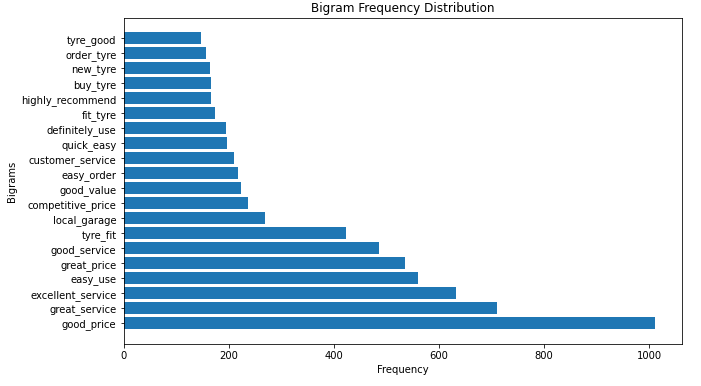Created by the author.

Trigram frequency distribution plot:

```trigram_data_items = trigram_count_dict.items()
trigram_data_list = list(trigram_data_items)
trigram_df = pd.DataFrame(trigram_data_list,columns=['word','count'])
plt.rcParams["figure.figsize"] = (10,6)
y=trigram_df['word']
x=trigram_df['count']
plt.barh(y, x)
plt.ylabel("Trigrams")
plt.xlabel("Frequency")
plt.title("Trigram Frequency Distribution")
plt.show()

```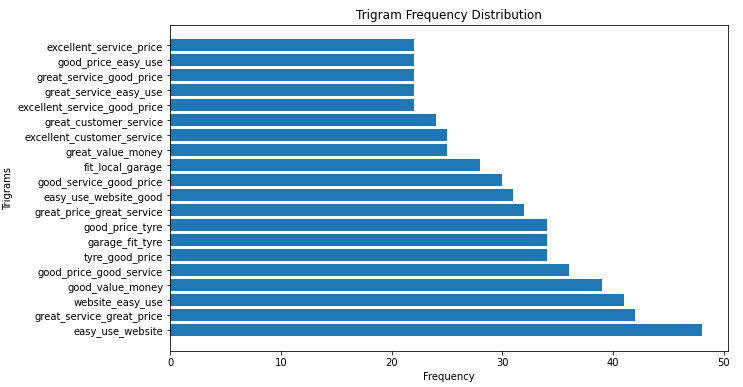Created by the author.

Visualization of the top 20 bigrams and top 20 trigrams wrt their frequency of occurrence provided me insight to use a list of token/bigrams of sentences rather than a list of token/trigram as most of the information inferred by trigrams phrases was already being conveyed by bigrams.

2. Retrieving noun and verb chunks from the list of tokens (bigrams) for each review document.

Noun and verb chunks carry the most useful information for conveying meaning in a summarized form for each document. In most cases, only noun chunks are enough to capture insights, but in this case, information like “delivery”, “wait”, etc. was getting lost when I chose only noun chunks. Thus, I used both the noun and verb chunks to capture as many insights as possible by keeping only the noun, adjective, proper noun, verb, and adverb tags from the parts of speech.

```def return_noun_and_verb_chunks(texts):
list_to_return = []
for sentence in texts:
doc3 = nlp(" ".join(sentence))
list_to_return.append([str(token) for token in doc3 if token.pos_ in pos_tags_to_keep])
return list_to_return
data_for_lda = return_noun_and_verb_chunks(data_bigrams)

```

### Corpus creation for LDA

A dictionary was made using a list returned from the function “return_noun_and_verb_chunks” defined in the code snippet presented above.

```from gensim.test.utils import common_texts
from gensim.corpora.dictionary import Dictionary
from gensim import corpora, models
def create_dictionary_for_lda(data_for_lda):
lda_dict = Dictionary(data_for_lda)
return lda_dict
lda_dictionary = create_dictionary_for_lda(data_for_lda)
lda_dictionary.filter_extremes(no_below=5)
####  This will remove all such tokens which are contained in less than 5 documents
texts = data_for_lda

```

This dictionary was then used to obtain a Bag of Words representation for each document in the corpus as this representation is required as a parameter by the gensim LDA model.

```bow_corpus = [lda_dictionary.doc2bow(text) for text in texts]

```

## GRID search for finding optimal number of topics

The LDA model requires an integer value for a hyperparameter called “num_topics”(i.e., number of topics). This is the most crucial hyperparameter that decides the number of requested latent topics to be extracted from the training corpus (in our case, “bow_corpus”). I plotted coherence vs. the number of topics plot on the result obtained from grid search and then decided to choose that value for the number of topics where coherence was highest just before taking the drop.

```from sklearn.model_selection import GridSearchCV
from sklearn.decomposition import LatentDirichletAllocation
import matplotlib.pyplot as plt

def perform_grid_Search():
coherence = []
topics = [20,24,28,32,36,40,42]
for k in topics:
print('Round: '+str(k))
lda_model_bow = gensim.models.ldamodel.LdaModel(corpus=bow_corpus,id2word=lda_dictionary,num_topics=k,
random_state=100,update_every=1,iterations=200,
chunksize=4096,passes=80,alpha='auto',eta = 0.1,
per_word_topics=True)
coherence_model_lda = coherence_model_lda = CoherenceModel(model=lda_model_bow, texts=data_for_lda,
dictionary=lda_dictionary, coherence='c_v')
coherence.append((k,coherence_model_lda.get_coherence()))
return coherence
coherence = perform_grid_Search() #### Perform grid search
### Visualize coherence vs topic number plotplt.rcParams["figure.figsize"] = (9,4)
plt.plot(*zip(*coherence))
plt.show()

```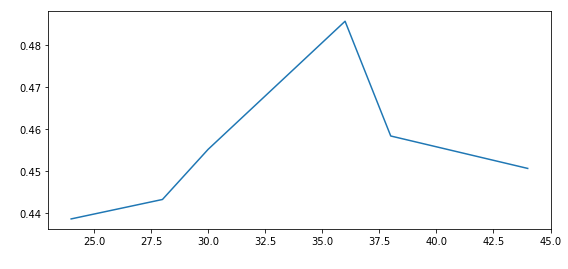y-axis: Coherence, and x-axis: Number of topics. Created by the author.

Thus I chose the number of topics = 36 (as it has the highest coherence before dropping).

### Fitting data to LDA model

There are a few other hyperparameters that I tuned while fitting the data to the LDA model:

1. Chunksize: This hyperparameter basically can make training faster (provided you have sufficient memory). I took a chunk size of 4096 (i.e., a power of 2 for efficient memory allocation).
2. ETA: This hyperparameter makes topics as close to a single word/bi-gram phrase as possible (i.e., word weight in each topic). The smaller the value of eta, the topic becomes closer and closer to a particular word (i.e., the weight of the particular word for that topic becomes higher than the weight of the same word for other topics that are far). I chose eta = 0.1 as it was returning the best coherence.
```lda_model_bow = gensim.models.ldamodel.LdaModel(corpus=bow_corpus,id2word=lda_dictionary,num_topics=36,
random_state=100,update_every=1,iterations=200,
chunksize=4096,passes=80,alpha='auto',eta = 0.1,
per_word_topics=True)

```

After fitting the data into the LDA model, I viewed the top 10 words present in each topic:

```for idx, topic in lda_model_bow.print_topics(-1):
print('Topic: {} \nWords: {}'.format(idx, topic))

```

As each topic returned by LDA is identified by an integer number, knowledge insight into the weight of the words/phrases present for each topic and what information does the documents that have those words/phrases capture gave me ample insights to assign a human-readable label for each topic number. Note that some of the topic numbers captured the same information. Thus, I assigned the same label clusters to such topics out of the 36 topics.

My many-to-many mapping for LDA returned the topic number and the human-readable label assigned accordingly looked like:

```#### 22 distinct topic clusters identified by many to many mapping
topic_equivalent_cluster_dictionary = {0:"Garage_service",
19:"Garage_service",
20:"service_duration",
1: "Value_for_money,Garage_service",
18:"Value_for_money,Garage_service",
26:"Value_for_money,Garage_service",
17:"Booking_confusion",
16:"Gararage_service,Location",
15:"Location",
14:"Value_for_money,Tyre_quality",
21:"Value_for_money,Tyre_quality",
22:"Discounts",
28:"Mobile_fitter",
29:"Booking_confusion,Change_of_date",
31:"Tyre_quality",
33:"Value_for_money,Ease_of_booking,Location",
35:"Value_for_money,Garage_service,Tyre_quality",
7:"Gararage_service,Ease_of_booking,Value_for_money",
6:"Delivery_punctuality",
23:"Delivery_punctuality",
4:"Change_of_date",
25:"Change_of_date",
34:"tyre_price",
3:"Wait_time,Length_of_fitting",
2:"Tyre_quality,Mobile_fitter",
13:"Value_for_money,Ease_of_booking",
24:"Value_for_money,Ease_of_booking",
12:"Delivery_punctuality,Value_for_money",
11:"Tyre_quality,Garage_service",
27:"Tyre_quality,Garage_service",
10: "Gararage_service,Ease_of_booking",
5: "Gararage_service,Ease_of_booking",
32: "Gararage_service,Ease_of_booking",
30:"Gararage_service,Ease_of_booking",
9:"Mobile_fitter",
8:"Mobile_fitter,Value_for_money"
}

```

Assignment of a topic number to each document present in the corpus was done on the basis of a probability distribution of topics present in the document, and the topic with the maximum probability was assigned to the document.

```import collections
from operator import itemgetter
def predict_topic_number_for_document(bow_corpus):
topics_predicted = []
for doc_bow_vector in bow_corpus:
topics_probability_score = lda_model_bow.get_document_topics(doc_bow_vector)
topic = max(topics_probability_score, key = itemgetter(1))
topics_predicted.append(topic)
topic_no_for_documents = predict_topic_number_for_document(bow_corpus)

```

Converting the topic number obtained for each document into a topic cluster using “topic_equivalent_cluster_dictionary” formed above:

```def get_topic_clusters(topic_no_for_documents):
topic_clusters = []
for topic_number in topic_no_for_documents:
topic_clusters.append(topic_equivalent_cluster_dictionary[topic_number])

topic_clusters = get_topic_clusters(topic_no_for_documents)
train_df['Label_cluster'] = topic_clusters ####assigning each document human readable topics cluster

```

The above code snippets completed the first sub-task out of two subtasks, as it returned topic clusters for each document. Now, learning a supervised model will make it feasible for a person to get a topic cluster for any new unseen document (provided the document is from the same domain).

## Supervised learning using BERT

Encoding each word present in the document with BERT embeddings provided rich contextual information for each labeled document present in the dataset. These embeddings were then passed onto a downward task of document classification.

```import tensorflow as tf
import tensorflow_hub as hub
import tensorflow_text as text
from official.nlp import optimization  # to create AdamW optimizer
from official.nlp import bert
from tensorflow import keras
from official.nlp import optimization  # to create AdamW optimizer
from official.nlp import bert

```

I used the original documents (without pre-processing the documents through spacy) and stored the labels (topic clusters) obtained from LDA for each corresponding document in a separate column in a CSV.

```train_df.head()

```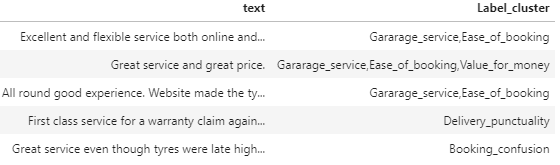The only pre-processing step I performed for this task was removing the non-ASCII characters, such as emojis etc., from the documents dataset.

```nlp = spacy.load("en_core_web_sm")
def remove_non_ascii(text):
doc = nlp(text)
to_return =  " ".join([str(token) for token in doc if token.is_ascii])
train_df['text'] = train_df.text.apply(remove_non_ascii)

```

Model selection:

```#### selecting BERT encoder having transformer layers(L) = 4,
#### dimension of o/p = 512 & no. of multi-headed attention = 8```
```tf_bert_encoder = 'https://tfhub.dev/tensorflow/small_bert/bert_en_uncased_L-4_H-512_A-8/1'

#### choosing pre-processor that is compatible with BERT encoder
#### selected
tf_bert_pre_process = 'https://tfhub.dev/tensorflow/bert_en_uncased_preprocess/3'
def build_classifier_model():
text_input = tf.keras.layers.Input(shape=(), dtype=tf.string, name='text')
preprocessing_layer = hub.KerasLayer(tf_bert_pre_process, name='preprocessing')
encoder_inputs = preprocessing_layer(text_input)
encoder = hub.KerasLayer(tf_bert_encoder, trainable=True, name='BERT_encoder')
outputs = encoder(encoder_inputs)
net = outputs['pooled_output']
net = tf.keras.layers.Dropout(0.1)(net)
net = tf.keras.layers.Dense(22, activation='softmax', name='classifier')(net)
#net = tf.keras.layers.Dense(1, activation=None, name='classifier')(net)
return tf.keras.Model(text_input, net)
classifier_model = build_classifier_model()

```

Assigning loss, evaluation metric, and optimization parameters:

```loss = tf.keras.losses.CategoricalCrossentropy(from_logits=True)
metrics = tf.metrics.CategoricalAccuracy()
epochs = 12
steps_per_epoch = 11370
num_train_steps = steps_per_epoch * epochs
num_warmup_steps = int(0.1*num_train_steps)
init_lr = 3e-5
optimizer = optimization.create_optimizer(
init_lr=init_lr,
num_train_steps=num_train_steps,
num_warmup_steps=num_warmup_steps,
)
classifier_model.compile(optimizer=optimizer,
loss=loss,
metrics=metrics)

```

Since the labels were English word phrases, I one-hot encoded them using the label binarizer provided by sklearn before fitting data into the model:

```from sklearn.preprocessing import LabelBinarizer
def get_encoded_labels(topic_clusters):
encoder = LabelBinarizer()
encoded_labels = encoder.fit_transform(topic_clusters)
return encoded_labels
labels = get_encoded_labels(train_df['Label_cluster'])

```

Fitting the model with a validation split of 15% in order to monitor model’s performance:

```history = classifier_model.fit(
train_df['text'],
labels,
validation_split=0.15,
epochs=epochs,
verbose=1
)

```

I obtained 92% accuracy on my validation set after 12 epochs. I hope your dataset gets even better results. I am looking forward to hearing any feedback or questions.

Original. Reposted with permission.

Bio: Saurabh Sharma is a Machine Learning Engineer with 3+ years of experience in formulating creative enterprise-level solutions, and is passionate about developing robust NLP algorithms

Related:

By subscribing you accept KDnuggets Privacy Policy

By subscribing you accept KDnuggets Privacy Policy

By subscribing you accept KDnuggets Privacy Policy